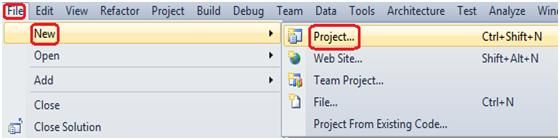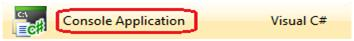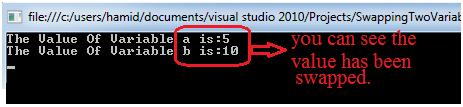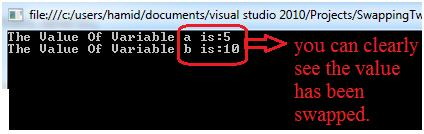1. Back To Blog

# .NET interview questions: - Different steps to swap two variable data without using third variable?

A .NET interview questions especially for fresher where the interviewer asks to check skills and many of the junior developer fails to answer this question, which is very sad.

So, let's create a sweet and simple example to see how exactly we can swap the two variables data without using third variable.

Before we go ahead and create an example of swapping two variables data let first see how we can swap two variable data using the third variable and later we will create an example for swapping two variables data without using third variable.

In order to see it practically you just need to follow the following steps.

Step1: - create a new Console Application for that just go to >> File >> New >> Project >> Windows >> Select Console Application.Step2: - Now simply just add the below code in to your program.cs file of your Console Application.

`class Programstatic void Main(string[] args)int a = 10;// Created int Variable a with value =10.int b = 5;// Created int Variable b with value =5.int temp = 0;// Creted a temp variable.temp = a;//Passing the value of a to temp.a = b;//passing the value of b to a.b = temp;//pasiing the value of temp to b.Console.WriteLine("The Value Of Variable a is:"+a);Console.WriteLine("The Value Of Variable b is:" +b);Console.ReadLine();}}`

In the above code snippet you can clearly see that I have used the third variable to swap the two variables values.

Now, simply just run your application and you will see the result like below diagram.Step3: - Now, let's see an example for swapping two variables value without using third variable.

Below is the code snippet for the same.

`static void Main(string[] args)int a = 10;// Created int Variable a with value =10.int b = 5;// Created int Variable b with value =5.a = a + b;b = a - b;a = a - b;Console.WriteLine("The Value Of Variable a is:"+a);Console.WriteLine("The Value Of Variable b is:" +b);Console.ReadLine();}}`

In the above code snippet you can clearly see that now I have not used the third variable temp to swap the value of two variables instead I have just added the few line of code like below code lines.

a = a + b;
b = a - b;
a = a - b;

Now, simply just run your application and will see the result like below diagram.See the following video on use of shadowing done in C#: -

Avail from the link more interview questions and answers for .NET  for preparation.

Regards,

Refer author's other blog for complete Most asked Dotnet interview questionsVisit us @ www.questpond.com or call us at 022-66752917... read more#### .NET interview questions 6th edition (Sixth edition) - By Shivprasad Koirala#### C# Design pattern interview questions with answers: - How Singleton is different from Static class?#### Exception Handling in C# using example step by step#### MVC vs ASP.NET ( MVC Interview question with answers )?#### What is the difference between each version of MVC 2, 3, 4, 5 and 6? (MVC Interview Questions)